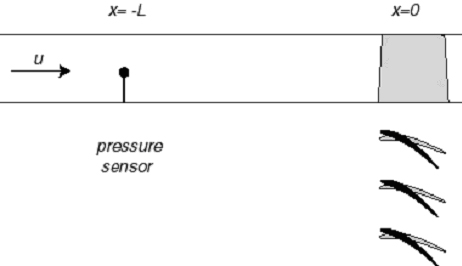# Exams

Both the midterm and final exams in 16.120 were conducted orally. Students were given the problems in advance, and alloted time to devise solutions. Each student would then present their solutions to the instructors. In contrast to a written exam, the format allows for dialog between faculty and student, often allowing more accurate evaluation.

## Final Oral Exam (also available in PDF)

Do both problems.

Problem 1.

Contrast small disturbance, linear, inviscid external supersonic flow with small disturbance, nonlinear, inviscid external hypersonic flow (no credit is given without clear explanations, perhaps bolstered by some equations).

Problem 2.

Consider a row of guide vanes in a compressible internal flow as shown below. There is a pressure probe at a measurement location a distance L upstream of the blade row. At time t=t0, the setting of the blades is changed suddenly such that they assume the position denoted by the solid blades, perturbing the mass flow in a one-dimensional sense.a) What are the governing equations representing the flow field in this situation?

b) Discuss the creation of flow field perturbations encountered in this situation and describe their attributes (an explanation is expected perhaps bolstered by some equations).

c) At what time does the pressure probe respond to the flow disturbance associated with the change in angle setting of the blades if: (1) the flow is subsonic, and (2) the flow is supersonic?

d) Sketch the propagation of the disturbances in an x-t diagram for both cases (1) and (2).

#### Learning Resource Types

assignment Problem Sets